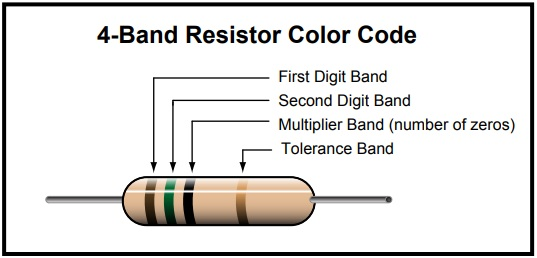# 4 Band Resistor Color Code Calculator

## How to read a typical 4-band resistor color code value

Reading the resistor from left to right, the first two color bands represent significant digits, the third band represents the decimal multiplier, and the fourth band represents the tolerance. Use our 4 Band Resistor Color Code Calculator online tool to calculate the information for color banded axial lead resistors of 4 band. Select the number of bands, then their colors to determine the value and tolerance of the resistors.

#### 4 Band Resistor Color Code Description

The number of bands is important because the decoding changes based upon the number of color bands. For the 4 band resistor:Four Bands are

Band 1 – First significant digit

Band 2 – Second significant digit

Band 3 – Multiplier

Band 4 – Tolerance

#### Formulae for calculating resistance of a 4-band resistor

Resistance = (First Significant Digit . Second Significant Digit) * Multiplier ± Tolerance %

First DigitSecond DigitMultiplierToleranceColor
00 zeroBlack
111 zeroBrown
222 zero2 %Red
333 zeroOrange
444 zeroYellow
555 zeroGreen
666 zeroBlue
777 zeroViolet
88naGrey
99naWhite
x 0.15% Gold
x 0.0110% Silver